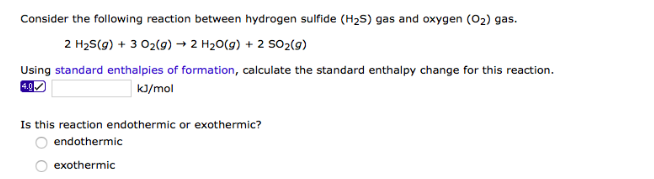# Problem: Consider the following reaction between hydrogen sulfide (H2S) gas and oxygen (O2) gas. 2 H2S(g) + 3 O2(g) → 2 H2O(g) + 2 SO2(g) Using standard enthalpies of formation, calculate the standard enthalpy change for this reaction. _____ kJ/mol Is this reaction endothermic or exothermic? a. endothermic b. exothermic

###### FREE Expert Solution
96% (335 ratings)###### Problem Details

Consider the following reaction between hydrogen sulfide (H2S) gas and oxygen (O2) gas.

2 H2S(g) + 3 O2(g) → 2 H2O(g) + 2 SO2(g)

Using standard enthalpies of formation, calculate the standard enthalpy change for this reaction.

_____ kJ/mol

Is this reaction endothermic or exothermic?

a. endothermic

b. exothermic